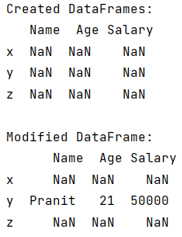# How to fill a DataFrame row by row?

Given a Pandas DataFrame, we have to fill it row by row. By Pranit Sharma Last updated : September 19, 2023

Pandas is a special tool which allows us to perform complex manipulations of data effectively and efficiently. Inside pandas, we mostly deal with a dataset in the form of DataFrame. DataFrames are 2-dimensional data structure in pandas. DataFrames consists of rows, columns and the data.

## Problem statement

Given a Pandas DataFrame, we have to fill it row by row.

## Filling a DataFrame row by row

DataFrame may consist of multiple columns and rows where each column may consist of NaN values. Now filling any of the row is a typical task. Pandas allows us to achieve this task by using pandas.DataFrame.loc property and then assigning a Series of elements.

Note

To work with pandas, we need to import pandas package first, below is the syntax:

```import pandas as pd
```

Let us understand with the help of an example,

## Python program to fill a DataFrame row by row

```# Importing pandas package
import pandas as pd

# Creating a DataFrame
df = pd.DataFrame(
columns=['Name','Age','Salary'],
index=['x','y','z']
)

# Display DataFrame with NaN values
print("Created DataFrames:\n",df,"\n")

# Adding data two second row
df.loc['y'] = pd.Series({'Name':'Pranit','Age':21,'Salary':50000})

# Display modified DataFrame
print("Modified DataFrame:\n",df,"\n")
```

### Output

The output of the above program is: